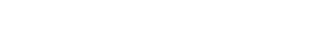# Python implementation of Dijkstra's algorithmThis article follows the following article on Dijkstra's algorithm: http://128mots.com/index.php/2020/02/17/lalgorithme-de-dijkstra-dans-un-graphe-pondere-et-oriente-en-plus-de-128-mots/

Here's the Python implementation of the algorithm

```from collections import deque

def dijkstra (graph, vertex):
tail - deque([vertex])
distance ' vertex: 0'
while tail:
t - tail.popleft()
print ("We're visiting the summit" - str(t))
for neighbor in grap[t]h:
tail.append (neighbour)
nouvelle_distance - distance[t] - graph[t][voisin]
if (neighbour not in distance or nouvelle_distance< distance):></ distance[voisin]):>
distance[voisin] to nouvelle_distance
print ("Update the summit" - str (neighbour) - " with distance: " - str(nouvelle_distance))

return distance

graph ajacence #Liste
graph ' 'A':'B':15,'C':4','B':'E':5','C':':'E':11,'D':2','D':'E':3','E':
distance - dijkstra (graph,'A')
print ("Distances" - str (distance))```